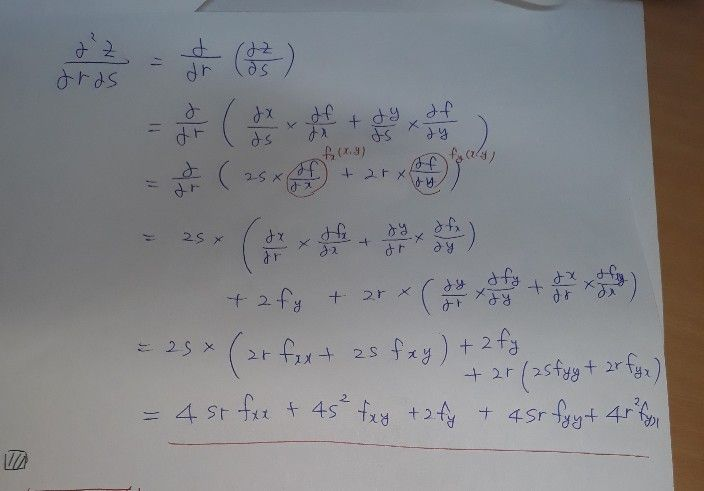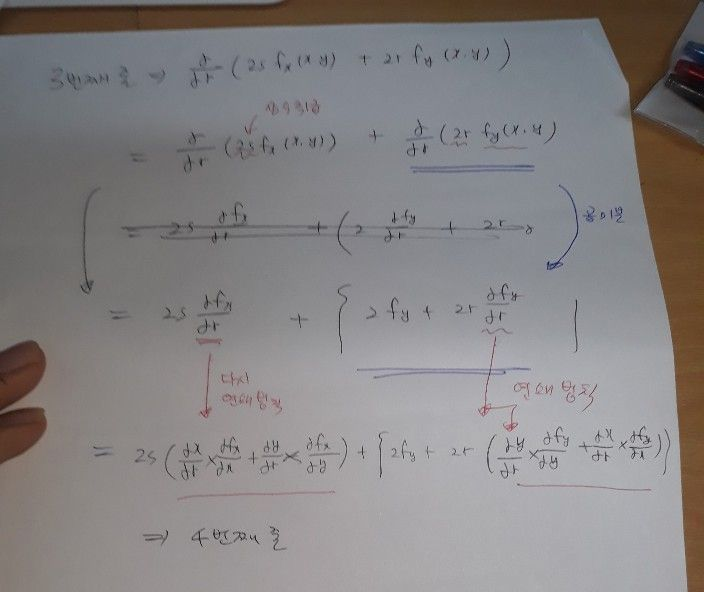수식부호
문제$z=f\left(x,y\right)$ 이고 $x=r^{2}+s^{2},y=2rs$ 일 때 $\dfrac {θ^{2}z} {θrθs}$ 를 구하라
고등학교
미적분
검색 수: 111
풀이콴다 선생님 - 파워업학생
4번째줄 설명점여콴다 선생님 - 파워업학생
감사요
아직도 궁금하다면?
콴다 선생님께 질문하기